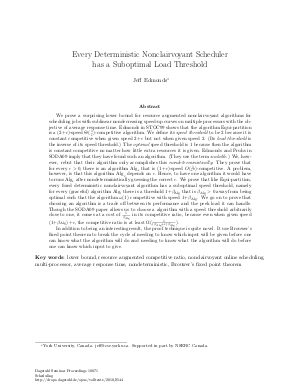Document# Every Deterministic Nonclairvoyant Scheduler has a Suboptimal Load Threshold

### Author Jeff Edmonds## File

DagSemProc.10071.6.pdf
• Filesize: 310 kB
• 21 pages

## Cite As

Jeff Edmonds. Every Deterministic Nonclairvoyant Scheduler has a Suboptimal Load Threshold. In Scheduling. Dagstuhl Seminar Proceedings, Volume 10071, Schloss Dagstuhl - Leibniz-Zentrum für Informatik (2010)
https://doi.org/10.4230/DagSemProc.10071.6

## Abstract

The goal is to prove a surprising lower bound for resource augmented nonclairvoyant algorithms for scheduling jobs with sublinear nondecreasing speed-up curves on multiple processors with the objective of average response time. Edmonds and Pruhs in SODA09 prove that for every $\e > 0$, there is an algorithm $\alg_{\e}$ that is $(1\!+\!\epsilon)$-speed $O({1 \over \e2})$-competitive. A problem, however, is that this algorithm $\alg_{\e}$ depends on $\e$. The goal is to prove that every fixed deterministic nonclairvoyant algorithm has a suboptimal speed threshold, namely for every (graceful) algorithm $\alg$, there is a threshold $1\!+\!\beta_{\alg}$ that is $\beta_{\alg} > 0$ away from being optimal such that the algorithm is $\Omega({1 \over \e \beta_{\alg}})$ competitive with speed $(1 \!+\! \beta_{\alg}) \!+\! \e$ and is $\omega(1)$ competitive with speed $1 \!+\! \beta_{\alg}$. I have worked very hard on it and have felt that I was close. The proof technique is to use Brouwer's fixed point theorem to break the cycle of needing to know which input will be given before one can know what the algorithm will do and needing to know what the algorithm will do before one can know which input to give. Every thing I have can be found at
• Scheduling

## Metrics

• Access Statistics
• Total Accesses (updated on a weekly basis)
0